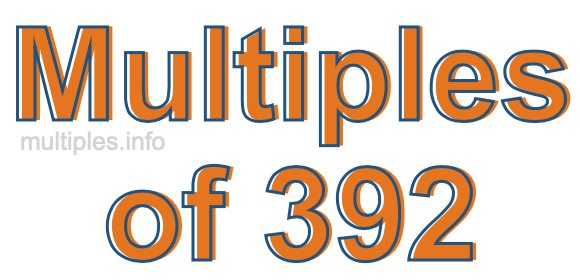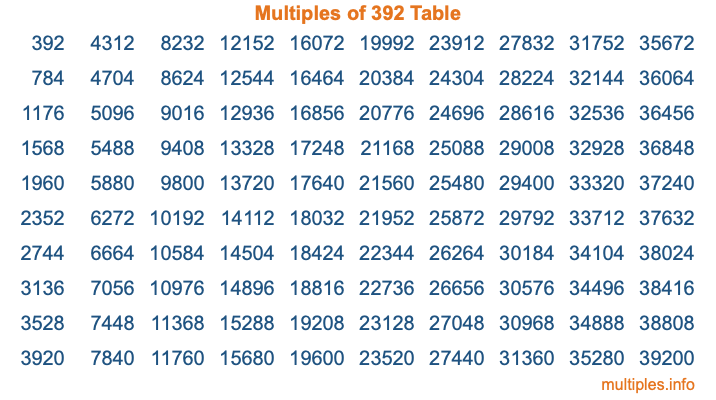Multiples of 392Welcome to the Multiples of 392 page. Here we will first teach you everything you will ever need to know about the multiples of 392, and then give you a study guide summary of everything we taught you to make sure you remember it all. Use this page to look up facts and learn information about the multiples of 392. This page will make you a multiples of three hundred ninety-two expert!

Definition of Multiples of 392
Multiples of 392 are all the numbers that when divided by 392 equal an integer. Each of the multiples of 392 are called a multiple. A multiple of 392 is created by multiplying 392 by an integer.

Therefore, to create a list of multiples of 392, you start with 1 multiplied by 392, then 2 multiplied by 392, then 3 multiplied by 392, and so on for as long as you want. Thus, the list of the first five multiples of 392 is 392, 784, 1176, 1568, and 1960. To see a larger list of multiples of 392, see the printable image of Multiples of 392 further down on this page. We also have a category where you can choose any nth multiple of 392.

Multiples of 392 Checker
The Multiples of 392 Checker below checks to see if any number of your choice is a multiple of 392. In other words, it checks to see if there is any number (integer) that when multiplied by 392 will equal your number. To do that, we divide your number by 392. If the the quotient is an integer, then your number is a multiple of 392.

Is  a multiple of 392?

Least Common Multiple of 392 and ...
A Least Common Multiple (LCM) is the lowest multiple that two or more numbers have in common. This is also called the smallest common multiple or lowest common multiple and is useful to know when you are adding our subtracting fractions. Enter one or more numbers below (392 is already entered) to find the LCM.

Check out our LCM Calculator if you need more details about the Least Common Multiple or if you need the LCM for different numbers for adding and subtraction fractions.

nth Multiple of 392
As we stated above, 392 is the first multiple of 392, 784 is the second multiple of 392, 1176 is the third multiple of 392, and so on. Enter a number below to find the nth multiple of 392.

th multiple of 392

Multiples of 392 vs Factors of 392
392 is a multiple of 392 and a factor of 392, but that is where the similarities end. All postive multiples of 392 are 392 or greater than 392. All positive factors of 392 are 392 or less than 392.

Below is the beginning list of multiples of 392 and the factors of 392 so you can compare:

Multiples of 392: 392, 784, 1176, 1568, 1960, etc.

Factors of 392: 1, 2, 4, 7, 8, 14, 28, 49, 56, 98, 196, 392

As you can see, the multiples of 392 are all the numbers that you can divide by 392 to get a whole number. The factors of 392, on the other hand, are all the whole numbers that you can multiply by another whole number to get 392.

It's also interesting to note that if a number (x) is a factor of 392, then 392 will also be a multiple of that number (x).

Multiples of 392 vs Divisors of 392
The divisors of 392 are all the integers that 392 can be divided by evenly. Below is a list of the divisors of 392.

Divisors of 392: 1, 2, 4, 7, 8, 14, 28, 49, 56, 98, 196, 392

The interesting thing to note here is that if you take any multiple of 392 and divide it by a divisor of 392, you will see that the quotient is an integer.

Multiples of 392 Table
Below is an image of the first 100 multiples of 392 in a table. The table is in chronological order, column by column. The first column has the first ten multiples of 392, the second column has the next ten multiples of 392, and so on.The Multiples of 392 Table is also referred to as the 392 Times Table or Times Table of 392. You are welcome to print out our table for your studies.

Negative Multiples of 392
Although not often discussed or needed in math, it is worth mentioning that you can make a list of negative multiples of 392 by multiplying 392 by -1, then by -2, then by -3, and so on, to get the following list of negative multiples of 392:

-392, -784, -1176, -1568, -1960, etc.

Multiples of 392 Summary
Below is a summary of important Multiples of 392 facts that we have discussed on this page. To retain the knowledge on this page, we recommend that you read through the summary and explain to yourself or a study partner why they hold true.

There are an infinite number of multiples of 392.

A multiple of 392 divided by 392 will equal a whole number.

392 divided by a factor of 392 equals a divisor of 392.

The nth multiple of 392 is n times 392.

The largest factor of 392 is equal to the first positive multiple of 392.

392 is a multiple of every factor of 392.

392 is a multiple of 392.

A multiple of 392 divided by a divisor of 392 equals an integer.

392 divided by a divisor of 392 equals a factor of 392.

Any integer times 392 will equal a multiple of 392.

Multiples of a Number
Here you can get the multiples of another number, all with the same attention to detail as we did for multiples of 392 on this page.

Multiples of
Multiples of 393
Did you find our page about multiples of three hundred ninety-two educational? Do you want more knowledge? Check out the multiples of the next number on our list!